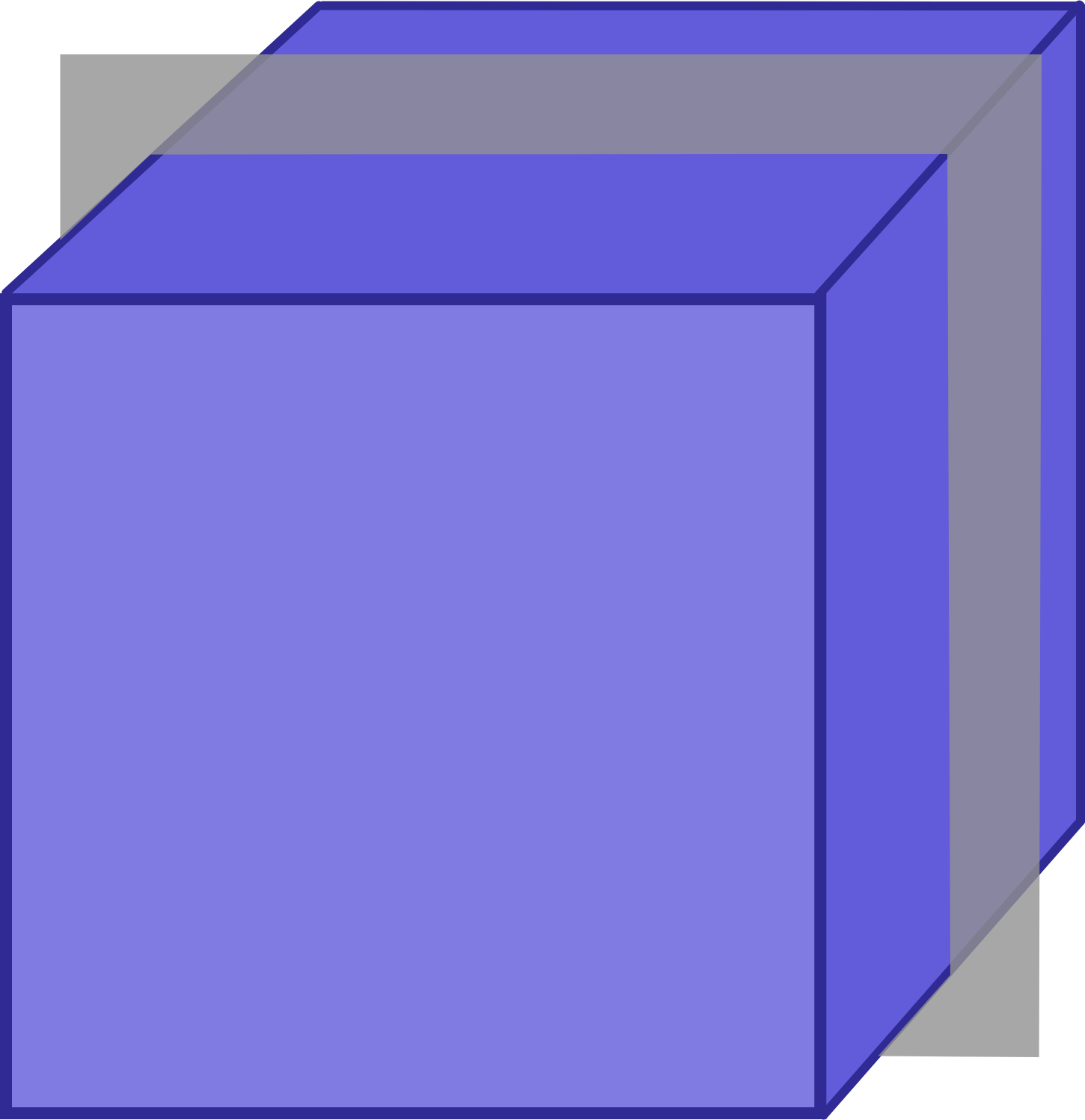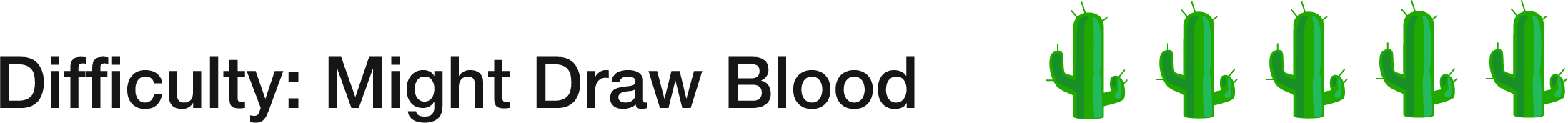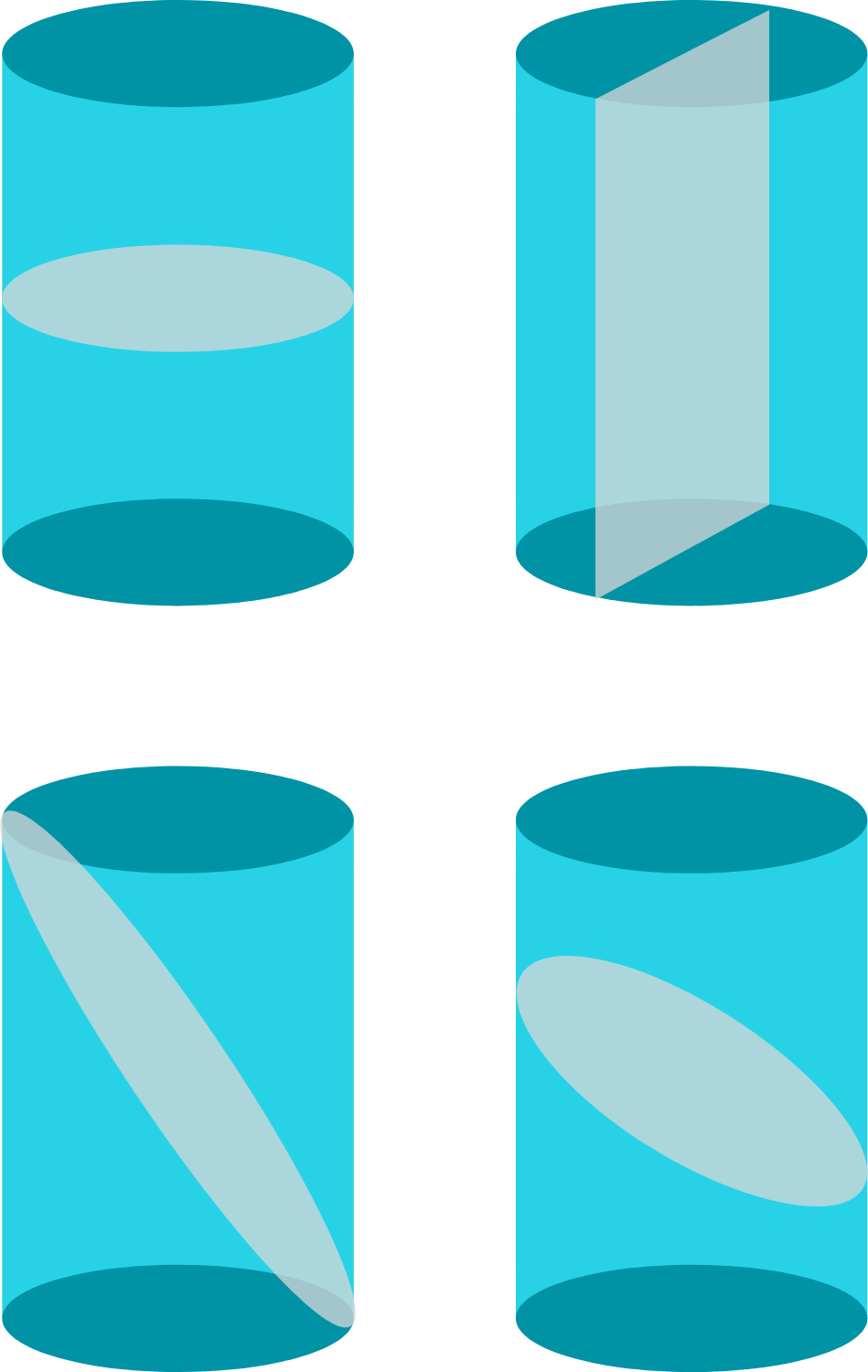# 94 of 100: Cross Sections

Geometry Level 3How many of the following 8 shapes can be created by taking a cross section of a cube?

 Equilateral Triangle Scalene Triangle Isosceles Triangle that is not equilateral Square Rectangle that is not a square Pentagon Hexagon OctagonA cross section is the resulting shape that's on the surface of the plane when a plane slices through a figure. Examples using a cylinder are shown below.×

Problem Loading...

Note Loading...

Set Loading...